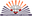Trending ▼   ResFinder# ICSE Class IX Prelims 2020 : Computer Applications (Daffodils Concept School (DCS), Sindhanur, Raichur)

3 pages, 29 questions, 0 questions with responses, 0 total responses,30Srinivas Siva Daffodils Concept School (DCS), Sindhanur, Raichur x AND puc M Tech(CS)
+Fave Message
 Home > srini23 >   F Also featured on: School Page icse9Formatting page ...

DAFFODILS CONCEPT SCHOOL ICSE Dec 19,2019 Class: IX Preparatory Examinations-I-2019-20 Sub: Computer Applications SECTION-A (Attempt all Questions) Time: 2hr Max. Marks: 100 Question 1: 1. What is the use of new operator? Give one example where new operator is used in java.  2. What are escape sequences? List the known sequences with their functions.  3. Write the size of the following integer data types both in terms of bytes and bits.  (a) int (b) short (c) long (d) byte 4. (a) Which package has defined the class system. (b) Write different types of comments with examples.  5. (a) The return type of the following functions: (i) Math.sqrt (ii) Math.round (b) Create an object cricket of class sports.  Question 2: 1. What is the difference between switch and if-else statement?  2. State the difference between: (i) = and == (ii) / and %  3. Write one similarity and one difference between (i) for and while loop (ii) while and do while.  4. What is token? Explain any two types of tokens with an example.  5. What do you mean by precedence of operators? Arrange the following operators given below in order of higher precedence to lower precedence: (i) && (ii) % (iii) <= (iv) ++  Question 3: 2( + )2 Write Java expression for (a) 2. (i) If a =5 and b=9; calculate the value of a+=a++ - ++b+a++%b++. (ii) What is the value of m after evaluating the following expression: m =9%++n+ ++n/2 When int m=10 ,n=6. Write the values stored in x and y if the following fragment code is executed. double a= 6.05; double b=14.74,c=18.3 double x=Math.abs(Math.ceil(a)); double y=Math.floor(Math.max( Math.ceil(b),Math.round(c)); What will be the out put of the following: int m=2,n=15; for(int i=1; i<5;i++); { m++; --n; } System.out.println( m= +m); System.out.println(n= +n); Find the output of the following segments, when (a) val=600 (b) val=1600 int val,sum,n=550; sum=n+val>1750?400:200 System.out.println(sum) Rewrite the following code segment in for loop. int y=55; while (y>0){ x=y%10; s=s+(x*10); y=y/10;} 3. 4. 5. 6. 2 (b) = ( + + ) 1. 2 3Formatting page ...Formatting page ...